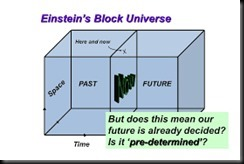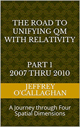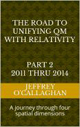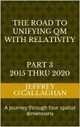# Is our universe expanding in space or is it expanding through time?

A few years after Albert Einstein unified space and time in his (and by now very well tested!) Theory of General Relativity he applied it to the entire universe and found something remarkable. The theory predicts that the whole universe is either expanding or contracting.

Later in 1929 the astronomer Edwin Hubble measured the velocities of a large selection of galaxies and found that the majority of them were moving away from us.  In other words, the universe was expanding.

However, is the universe expanding in space or is it expanding through time?

To answer this one must first define what time and space are.

Some define time only in the abstract saying that is an invention of the human consciousness that gives us a sense of order, a before and after so to speak.  To physicist’s it is a measure of the relative interval between events which is measured in units of time such as seconds minutes or hours.

However, space can be defined as the arena where events occur.  We use the measurements of inch or meter to define the position of those event in that arena.

As was mentioned earlier, Einstein’s General Theory of Relativity mathematically define the universe in terms of a melding of time with space.  However, as was mention above they are they have vastly different properties.  For example, one is measure in terms of second while the other is in inches or meters.

Therefore, it is very difficult to understand how time which is measured in seconds can have a dynamic effect on space measured in meters.

To this end Marina Corts, a cosmologist from the Royal Observatory, Edinburgh came up to what has come to be called the block universe.

Basically, it asks us to imagine a regular chunk of cement. It has three dimensions but we live in four dimensions: the three spatial dimensions plus one time dimension. A block universe is a four-dimensional block, but instead of being made of cement, it is made of space-time. And all of the space and time of the Universe are there in that block.”We can’t see this block, we’re not aware of it, as we live inside the cement of space-time. And we don’t know how big the block universe we live in is: “We don’t know if space is infinite or not. Or time – we don’t know whether it has a beginning or if it will have an end in the future. So, we don’t know if it’s a finite chunk of space-time or an infinite chunk.”

However, picture this presents a problem for cosmologists because if the merging of space and time causes it to become as ridge as a block of cement how can its spatial component be expanding.

It should be remembered only the spatial component of the universe is expanding not time.

Additionally, because Einstein defined the universe in terms of only four dimensions, one time and three spatial how can we understand its spatial expansion without adding an additional one because a spatial one cannot expand to one made up of time because, as mentioned earlier they have vastly different properties.

Yet, Einstein gave us an alternative when he defined the mathematical relationship between space and time in terms of the constant velocity of light because in doing so, he provided a method of converting a unit of time in a space-time environment to its equivalent unit of space in four *spatial* dimensions.  Additionally, because the velocity of light is constant, he also defined a one to one quantitative and qualitative correspondence between his space-time universe and one made up of four *spatial* dimensions.

In other words, Einstein’s mathematics actually defined two mathematically equivalent physical models of the universe one consisting of four-dimensional space-time and one of only four spatial dimensions.

Yet, because both of these models are mathematically equivalent and since we cannot physically observe  either a time or a fourth *spatial* dimension, we must look to the effects they would have on the ones we can observe to determine which one of these physical models is correct.

For example, if we were a two-dimensional creature living on the surface of a balloon that was inflating, we could explain its spatial expansion by assuming we were living in an environment consisting three spatial dimensions because they have the same properties as the two dimension surface of the balloon therefore, it could expand through it.  However, we could not explain it by assuming that we were living in an environment consisting of only time and the two-dimensional surface of the balloon because time as mentioned earlier it does not have the properties of space and therefore could not expand in it.

Similarly, we can explain why our three-dimensional world was undergoing a spatial expansion by assuming we were living in an environment or universe consisting four *spatial* dimensions because it would have the same spatial properties as the three dimension one we live in.  However, we could not if we assume our universe consisted of four-dimensional space-time because time does not have the properties of space and therefore similar to the surface of the balloon it could not expand in it.

As was mentioned earlier  “A few years after Albert Einstein unified space and time (and by now very well tested! ) in his theory of General Relativity” and showed it can be “applied to the entire universe .” Therefore, he also showed that because of their mathematical equivalence, a physical model based on one unifying three-dimensional space with a fourth *spatial* dimension has also been very well tested and could also be applied it to the entire universe.

However, as was shown above his physical model based on four *spatial* dimensions pass an additional test which his space-time model cannot, that of explaining the spatial expansion of our three-dimension environment.

Later Jeff

 The Road to Unifying QM with Relativity part 1 2007 thru 2010Ebook \$8.00Paper Back \$15.00 The Road to Unifying QM with Relativity part 2 2011 thru 2014Ebook \$8.00Paper Back \$16.00 The Road to Unifying QM with Relativity part 3 2015 thru 2020Ebook \$8.00Paper Back \$18.00